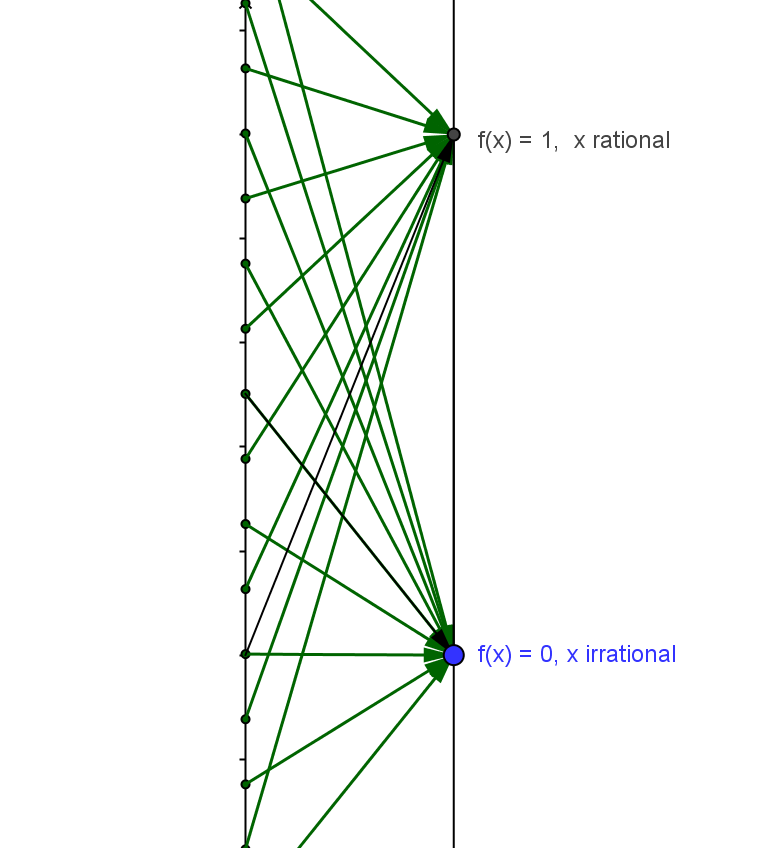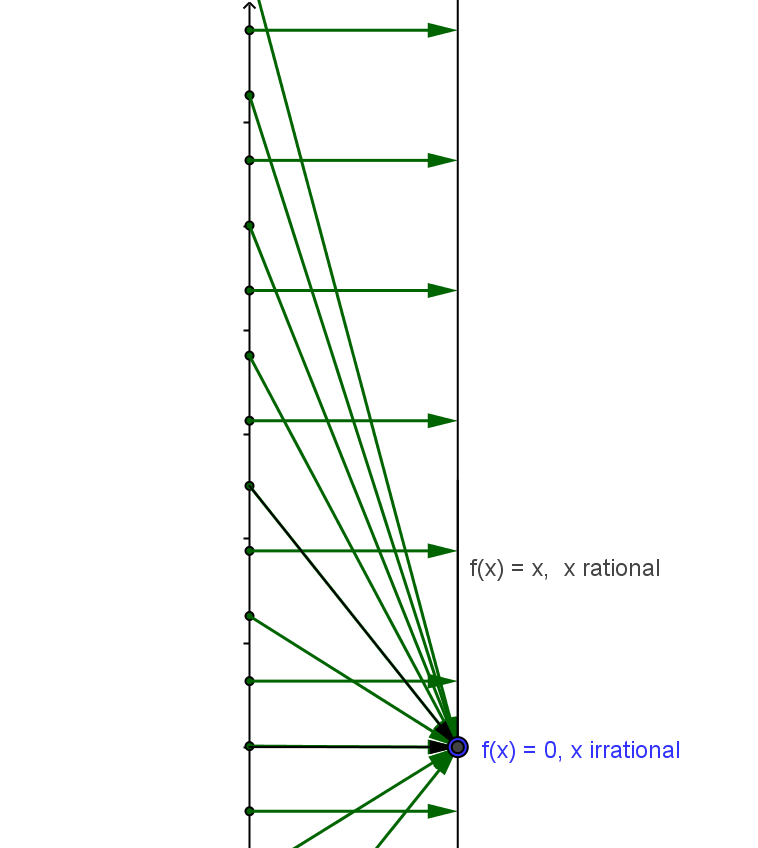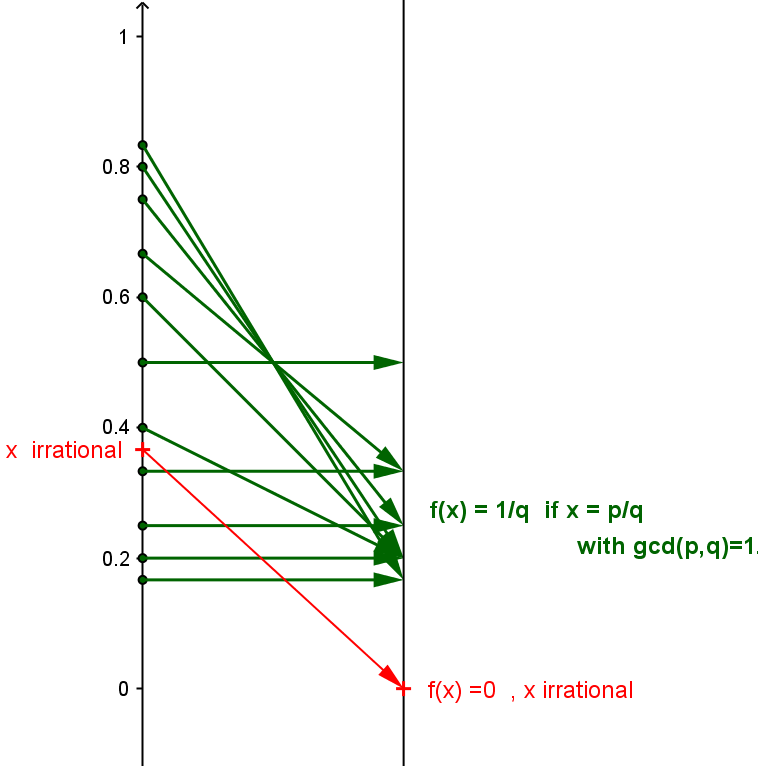CCD.DLC. Example.5.  Discontinuities Almost Everywhere.
Functions that have a definition that specify a value at each number without an algebraic rule for any interval can be problematic and hard to visualize in a technology that is bound by the use of rational numbers for locating points on a screen. These functions can fail to be continuous at any number because there is no possible limit of the function at any number. In other words these functions fail to be continuous at any number $a$ because there is no number $L$ where $\lim_{x \to a} f(x) = L$.

One of the simplest such functions is $f(x) = 1$ when $x$ is a rational number while $f(x) =0$ when $x$ is an irrational number. For any $a$ and $\epsilon =1/2$, there is no $\delta>0$ for which for any $x$ if $|x-a|<\delta$ then $|f(x)-f(a)|< 1/2$ because in any interval there are both rational and irrational numbers.
So $f$ is not continuous at any number. On a graph or mapping diagram created using technology, only the rational numbers can be used for the domain and so the function $f$ would appear to be the constant function $g(x)=1$ for all $x$
One can illustrate this function by creating diagrams by hand with selected values to indicate what is intended by the definition.

Such examples are used to illustrate functions that are not continuous at any single point $a$ because  $\lim_{x \to a} f(x)$ does not exist.

There  are variations on this theme that provide different sets of numbers where the function is not continuous.One such function is $f(x) = x$ when $x$ is a rational number while $f(x) =0$ when $x$ is an irrational number. For any $a\ne 0$ and $\epsilon = \frac a2$, because in any interval there are both rational and irrational numbers, there is no $\delta>0$ for which for any $x$ if $|x-a|<\delta$ then $|f(x)-f(a)|< \frac a2$.
So $f$ is not continuous at any number, $a \ne 0$ However this function is continuous at $a = 0$. [Proof left to the reader.] On a graph or mapping diagram created using technology, only the rational numbers can be used for the domain and so the function $f$ would appear to be the function $g(x)=x$ for all $x$
Again one can illustrate this function by creating diagrams  by hand with selected values to indicate what is intended by the definition.Another particularly remarkable example is  $f(x) = \frac 1q$ when $x$ is a rational number of the form $\frac pq$ where $p$ and $q (q>0)$ are relatively prime integers while $f(x) =0$ when $x$ is an irrational number.
For this function, $f$ is continuous at every irrational number but not continuous at any rational number.
Again one can illustrate this function by creating diagrams  by hand with selected values to indicate what is intended by the definition and why the function is continuous at any irrational number.